info@biomedres.us   +1 (720) 414-3554
One Westbrook Corporate Center, Suite 300, Westchester, IL 60154, USA

# Biomedical Journal of Scientific & Technical Research

June, 2021, Volume 36, 3, pp 28477-28479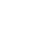Mini Review

Mini Review

# On the Effect of the Variable Electromagnetic Field on the Movement of Red Blood Cells on the Circulatory System

Author Affiliations

Moscow Aviation Institute (National Research University) (MAI), Russia

Received: May 20, 2021 | Published: June 09, 2021

Corresponding author: SO Gladkov, Moscow Aviation Institute (National Research University) (MAI), Volokolamskoe shosse, 4. 125993. Moscow, Russia

DOI: 10.26717/BJSTR.2021.36.005843

#### Abstract

In the paper analyzed the movement of red blood cells through blood channels while considering the effects of external electromagnetic fields on them. It is shown that the external variables of EM fields strongly affect the internal structure of red blood cells up to their destruction.

Keywords: Red Blood Cells; Variable Electromagnetic Fields; Hydrodynamic Current

#### Mini Review

In this paper, we will conduct a detailed analysis of the problem, the essence of which is the following. The flow of blood through the blood channels is considered, provided that the biological object is exposed to external influences in the form of a variable electromagnetic field (short for EM). At the same time, the current is purely hydrodynamic, and the blood flow itself is a continuum with viscosity η . The main equations of motion are the Navier- Stokes’s equations and the continuity equation, but the effect on the charged red blood cells of the outer variable EM field must also be considered.

The energy of the interaction of moving charged particles with the EM field can be represented in the form [1-3].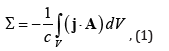where c − is the speed pf the light, V − is the volume of the channel, current density is determined only by red blood cells and can be recorded as = ρq j v, where q ρ − is the density of charged particles, v − speed pf the flux, A − and is the magnetic potential. Therefore, the voluminous force that works on some dedicated ensemble of red blood cells can be determined by the ratio of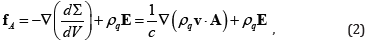where E− resulting from magnetic induction, the electric field (see also the works [4-6]).

Considering the density of charges homogeneous, hence it is easy to get for bulk strengthwhere B = rotA − is the magnetic field induction.

With the account (3) the Navier-Stokes equation can be written down aswhere ρ − volumes blood density.

If the speed flow is homogeneous at account the expr. (3), we get out of the equation (4)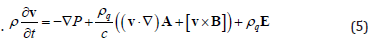Consider the case when the heterogeneity of the magnetic field δ r exceeds the average diameter of the blood channel d (i.e. δ r  d ). Then the magnetic field can be considered homogeneous and choose the dependence of magnetic potential in the form (see )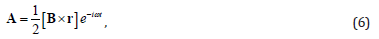where r − is a radius – vector, conducted from the beginning of the coordinates to the observation point, ω − is a frequency field. Therefore, the electric field will be followingAs a result, the equation (5) becomesChoosing the channel’s axis as an axis z , and the direction of the magnetic field along the axis y , we find from (8)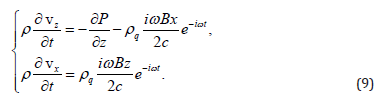Because the speed of cell bloods is oriented along the hydrodynamic current lines, i. e. vz = , vx =  z x out (9) immediate follows the system of equationswhere parameterWhere it is easy to find an equation at the coordinate z describing the rate of flow of red blood cells vz = z through the channel under the influence of two factors - pressure gradient and variable EM fields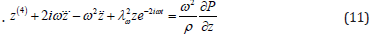The equation (11) describes a rather complex oscillating movement of blood through the vessels in the conditions of exposure to variable EM fields under the influence of a constant pressure gradient. As can be seen from (11), blood flow can change its direction very much, which in certain conditions will have a negative impact on the whole body. The most significant negative impact on the biological object will create a high-frequency EM oscillation. When exposed to a separate red blood cell can occur its destruction, provided that the surface tension will not withstand the resonant load from the external EM field and break. The critical value of this field is easy to estimate if we use of the following considerations. Surface energy of spherical red blood cell isThe equation of fluctuations in the internal charge of red blood cell can be described as an oscillator, which is under the influence of a variable field. It means that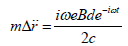From where the speed of the oscillations is the following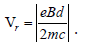So the kinetic energy of rocking is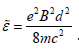Comparing (12) and (13) come to the condition of destruction of red blood cellBy order of magnitude, this critical field is about 107 ýðñò åä .

In conclusion of this message we note.

1. The strong effect of variable EM fields on the movement of red blood cells in the blood is shown.

2. The amplitude of the critical magnetic field, in which red blood cells are destroyed is assessed.

Mini Review

# On the Effect of the Variable Electromagnetic Field on the Movement of Red Blood Cells on the Circulatory System

Author Affiliations

Moscow Aviation Institute (National Research University) (MAI), Russia

Received: May 20, 2021 | Published: June 09, 2021

Corresponding author: SO Gladkov, Moscow Aviation Institute (National Research University) (MAI), Volokolamskoe shosse, 4. 125993. Moscow, Russia

DOI: 10.26717/BJSTR.2021.36.005843

#### Abstract

In the paper analyzed the movement of red blood cells through blood channels while considering the effects of external electromagnetic fields on them. It is shown that the external variables of EM fields strongly affect the internal structure of red blood cells up to their destruction.

Keywords: Red Blood Cells; Variable Electromagnetic Fields; Hydrodynamic Current